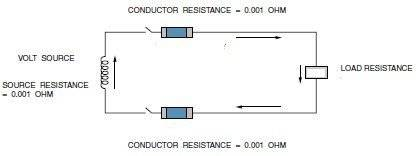# Calculating fuse size, current and voltage in a circuit

• Engineering
Jon Canine(a) Calculate the size of Conductor and Fuse, the amount of Current through the conductor, Voltage ACROSS the existing LOAD and power consumed by the EXISTING LOAD under normal circuit condition (when the switch of the above circuit will be closed).

(b) Calculate the overload current, voltage and power if additional two 30 OHMS loads are connected in parallel across the existing load. (When the switch will be closed).

(c) Calculate the short circuit current, voltage and power if the LOAD is shorted. (When the switch will be closed).

(d) What will happen to this circuit if HOT” CONDUCTOR COMES IN CONTACT WITH METAL RACEWAY OR OTHER METAL OBJECT.

(e) What will happen to this circuit under open circuit condition? Calculate the open circuit current, voltage and power if one 30 OHMS load IS connected across the existing load under this open circuit condition? (When the switch will not be closed).

I = E/R

## The Attempt at a Solution

[/B]
I know:

240 = 7.992 or 8amps.
24 + 0.001 + 0.001 + 0.001

How do i figure out fuse size and voltage?

i know this is very basic... Any help is greatly appreciated.

Gold Member
2021 Award
I don't see any fuse in the drawing. Do you understand what fuses DO? What do you need to know to know what "size" fuse you need?

Mentor
@phinds, I figure those blue capped rectangles are meant to be line fuses.

The problem statement is incomplete as it doesn't specify the source voltage or the load resistance. However, judging by the attempt at solution clues (I say clues because they aren't proper equations) I'm guessing that the source voltage is 240 V and the load resistance is 24 Ω.

We also don't know the length of the wiring, so determining the conductor size (assuming copper) from its given resistance is not possible. Of course, this may be some sort of Electrical Code test question where a normal residential circuit is assumed and wire gauge is determined by the load current.

Last edited:
Gold Member
2021 Award
@phinds, I figure those blue capped rectangles are meant to be line fuses.
Yeah, I thought that might be the case but since the label said "conductor resistance" I took them to be resistors representing the conductor resistance.

The problem statement is incomplete as it doesn't specify the source voltage or the load resistance. However, judging by the attempt at solution clues (I say clues because they aren't proper equations) I'm guessing that the source voltage is 240 V and the load resistance is 24 Ω.

We also don't know the length of the wiring, so determining the conductor size (assuming copper) from its given resistance is not possible. Of course, this may be some sort of Electrical Code test question where a normal residential circuit is assumed and wire gauge is determined by the load current.
You're probably right ... I just have an antipathy to inadequate problem statements.

Jon Canine
damn... sorry. this part didn't paste:

Given that the VOLTAGE OF THE SOURCE =240V, LOAD RESISTANCE = 30 OHMS

Mentor
Jon, can you clarify the nature of the question? Is it an Electrical Code or Standards type question? Otherwise I don't see enough information to be able to determine a conductor size.

Gold Member
2021 Award
damn... sorry. this part didn't paste:

Given that the VOLTAGE OF THE SOURCE =240V, LOAD RESISTANCE = 30 OHMS
And you still have not answered the questions I asked you in post #2.

Jon Canine
This is just an electrical installations course. @phinds, you are correct - the fuses would be the blue rectangles by the switches. I understand what fuses do and I thought I needed: I (Amps) = P (Watts) ÷ V (Voltage) to figure it out...

Gold Member
2021 Award
This is just an electrical installations course. @phinds, you are correct - the fuses would be the blue rectangles by the switches. I understand what fuses do and I thought I needed: I (Amps) = P (Watts) ÷ V (Voltage) to figure it out...
Well, I suppose you could do it that way, but I don't see why you would want to do it the long way by computing power when you can just use ohms law directly

Mature student
View attachment 78635

(a) Calculate the size of Conductor and Fuse, the amount of Current through the conductor, Voltage ACROSS the existing LOAD and power consumed by the EXISTING LOAD under normal circuit condition (when the switch of the above circuit will be closed).

(b) Calculate the overload current, voltage and power if additional two 30 OHMS loads are connected in parallel across the existing load. (When the switch will be closed).

(c) Calculate the short circuit current, voltage and power if the LOAD is shorted. (When the switch will be closed).

(d) What will happen to this circuit if HOT” CONDUCTOR COMES IN CONTACT WITH METAL RACEWAY OR OTHER METAL OBJECT.

(e) What will happen to this circuit under open circuit condition? Calculate the open circuit current, voltage and power if one 30 OHMS load IS connected across the existing load under this open circuit condition? (When the switch will not be closed).

I = E/R

## The Attempt at a Solution

[/B]
I know:

240 = 7.992 or 8amps.
24 + 0.001 + 0.001 + 0.001

How do i figure out fuse size and voltage?

i know this is very basic... Any help is greatly appreciated.

I have the same question, however it supplied the voltage at 120 and a load resistance of 20 OHMS. Also, it says to consider that the length of both, black and red hot conductor is 50 feet. I calculated 5.99910 amperes so 6 amps but not sure what to do next? Any ideas

Gold Member
2021 Award
Any ideas